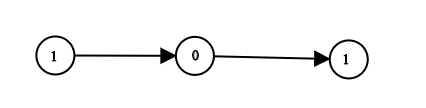1290. Convert Binary Number in a Linked List to Integer

Given `head` which is a reference node to a singly-linked list. The value of each node in the linked list is either 0 or 1. The linked list holds the binary representation of a number.

Return the decimal value of the number in the linked list.

Example 1:```Input: head = [1,0,1]
Output: 5
Explanation: (101) in base 2 = (5) in base 10
```

Example 2:

```Input: head = 
Output: 0
```

Example 3:

```Input: head = 
Output: 1
```

Example 4:

```Input: head = [1,0,0,1,0,0,1,1,1,0,0,0,0,0,0]
Output: 18880
```

Example 5:

```Input: head = [0,0]
Output: 0
```

Constraints:

• The Linked List is not empty.
• Number of nodes will not exceed `30`.
• Each node's value is either `0` or `1`.

1290. Convert Binary Number in a Linked List to Integer
``````struct Solution;
use rustgym_util::*;

impl Solution {
let mut res = 0;
while let Some(n) = p {
res *= 2;
res += n.val;
p = &n.next;
}
res
}
}

#[test]
fn test() {
let head = list!(1, 0, 1);